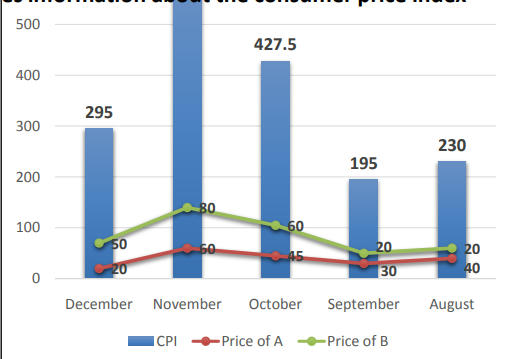# Data Interpretation (Set-59) | Maths & Reasoning | Free PDF Download

In a country, the consumer price index (CPI) is calculated at the beginning of each month on the basis of the prices of the two commodities i.e. A and B. If pA (in Rs.) and pB (in Rs.) denotes the price of commodity A and B respectively, then CPI = a x pA + b x pB, where a and b are the weights given to the commodities A and B respectively and these weights remain constant every month. The line graph below provides information about the price of the two different commodities in different months of the year. And the bar graph below provides information about the consumer price index in different months.1) Had the weights given to commodity A and commodity B been increased by 20% and 50% respectively, then find the difference between the actual CPI and new CPI in the month of October.

1. a) 154.5 b) 166.5 c) 186.5 d) 197 e) None of these 295

A shopkeeper has three bags P, Q and R, and each bag contains Toffees of three different colors i.e. Red, Yellow and White. The ratio of the number of Toffees in bag P, Q, and R is 9:x:11 respectively, and the number of Toffees in bag R is 30 more than the number of Toffees in bag Q. The ratio of the number of yellow Toffees to white Toffees in bag P is 5:6 respectively, and the probability of choosing a red Toffee from bag P is 4/15. The number of red Toffees in bag Q is one-third of the total number of red Toffees in bag P and bag R taken together. The probability of choosing a yellow Toffee from bag Q is 1/5. The number of red Toffees in bag R is equal to the number of white Toffees in bag P, and the probability of choosing a white Toffee from bag R is 3/11. Total number of Toffees in bag Q is 25% of the total number of Toffees of bag P and bag R taken together. 5) If some white toffees are transferred from bag P to bag Q such that probability of drawing a white toffee from bag Q is increased by 0.1, then find the number of white toffees remaining in bag P after the transfer of toffees.

1. 9 b) 12 c) 10 d) 13 e) 15 6) Find the average number of White toffees in each bag? a) 13 b) 14 c) 15 d) 16 e) None of these